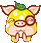登录 注册# 《刀剑英雄》 合成暗金材料公式大全

 青夹叶=血玉碎片+青玉碎片 　　黄 苓=青夹叶 +雨花石 　　生血茸=黄 苓 +雨花石 　　祝余草=生血茸+青荚叶 =生血茸+通灵石 　　戒精草=青荚叶+黄苓 =青荚叶+通灵石 　　槲藤根=黄苓+生血茸 =黄 苓+通灵石 　　人参果=祝余草+生血茸+青荚叶 =祝余草+五瓣翠绿镯 　　铁线兰=戒精草+青荚叶+黄 苓 =戒精草+五瓣翠绿镯 　　鹿活草=槲藤根+黄 苓+生血茸 =槲藤根+五瓣翠绿镯 　　金莲子 =人参果+祝余草+生血茸+破损鸡血石 =人参果+飞羽镯 　　冰髓精浆 =铁线兰+戒精草+青荚叶+通灵石 =铁线兰+飞羽镯 　　七节太阳竹=鹿活草+槲藤根+黄 苓 =鹿活草+飞羽镯 　　初阳温火符=冰种玉碎片+紫水晶碎片 　　桃木钉=初阳温火符+白玉碎片=玲珑花+小羽花石 　　犬头钉=桃木钉+初阳温火符+破损白玉=桃木钉+龟背 　　镇狱钉=犬头钉+桃木钉+初阳温火符=犬头钉+蛟皮护手 　　青龙钉=镇狱钉+犬头钉+白玉=镇狱钉+五行旗木

《刀剑英雄2》修罗优缺点及成长之路

《刀剑英雄2》各种技能属性解析